﻿ 基于集成学习的红外双波段被动测距模型 Passive IR Ranging Based on Ensemble Learning

Computer Science and Application
Vol. 09  No. 03 ( 2019 ), Article ID: 29324 , 9 pages
10.12677/CSA.2019.93068

Passive IR Ranging Based on Ensemble Learning

Zhao Jing*, Xiaoning Fu

College of Electromechanical Engineering, Xidian University, Xi’an Shannxi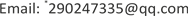Received: Feb. 25th, 2019; accepted: Mar. 13th, 2019; published: Mar. 20th, 2019ABSTRACT

To improve the practicability and accuracy of passive IR ranging, a new passive IR ranging method based on ensemble learning is proposed. The atmospheric transmittance data of 3.5 um - 4.0 um and 4.5 um - 4.7 um in different range are generated by MODTRAN, then these data are converted to voltage based on infrared transmission theory, using these voltages to establish single gbdt, xgboost and adaboost models under the framework of boosting, and then merge the three models with stacking method as the final model. At last, the accuracy of the model is tested by testing data. For comparison, average method is also used to merge the three models above. The result shows that stacking method can make further improvement of accuracy.

Keywords:Passive IR Ranging, Dual-Band, Infrared Radiation, Ensemble LearningCopyright © 2019 by author(s) and Hans Publishers Inc.1. 引言

2. 红外被动测距原理

${L}_{bb}\left(\lambda ,T\right)={\int }_{{\lambda }_{1}}^{{\lambda }_{2}}\frac{C1}{{\lambda }^{5}}\left(\frac{1}{{e}^{\frac{c2}{\lambda T}}-1}\right)\text{d}\lambda$ (1)

${E}_{R}=\tau \left(\lambda ,R\right)\frac{{L}_{bb}\left(\lambda ,T\right)}{{R}^{2}}$ (2)

$\tau \left(\lambda ,R\right)={e}^{-\mu \left(\lambda \right)R}$ (3)

$\mu \left(\lambda \right)$ 为大气的消光系数，由吸收系数和散射系数组成，消光系数和大气的气象条件密切相关。大气透过率 $\tau \left(\lambda ,R\right)$ 是一个与波长、距离气象条件等因素相关的量，难以直接计算，该数据可由MODTRAN软件仿真生成。

${I}_{sensor}=\tau \left(\lambda ,R\right)\frac{{L}_{bb}\left(\lambda ,T\right)}{{R}^{2}}\cdot {A}_{s}\cdot {r}_{I}$ (4)

3. 集成学习算法

Bagging  是一种并行的集成学习方法，它首先对原始训练数据集进行有放回的抽样，从而得到多份数据集，然后对每一份数据集训练一个基模型，这些基模型彼此是独立的，最后采用投票法(分类问题)或平均法(回归问题)，将多个基模型的输出结果融合起来得到最终的输出。Boosting  则是一种串行的训练策略，它每轮迭代生成的模型都和前一轮的模型有一定的关系，最终的模型为一系列模型的线性组合，基于boosting架构的方法有GBDT、xgboost、adaboost等。

Xgboost的全称为extreme Gradient Boosting，它是由陈天奇  提出的在GBDT基础上的一种改进算法，利用了损失函数的一阶、二阶导信息，同时为了防止过拟合，在损失函数中引入了正则化项。Xgboost引入了并行化的训练方法，相对于gbdt有着更快的训练速度。

4. 训练数据的获取与处理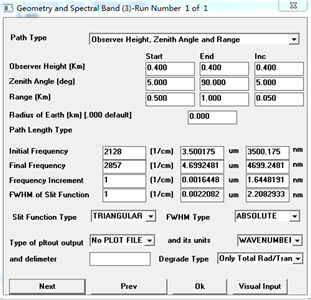Figure 1. The setting of MODTRAN

5. 本文建模的流程与预测结果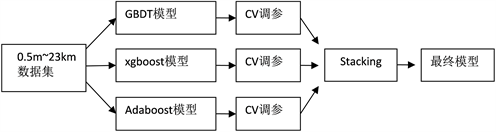Figure 2. The flow chart of stacking model

$\phi \left({F}_{i}\left(x\right)，{Y}_{i}\right)=\frac{{\left({Y}_{i}-{F}_{i}\left(x\right)\right)}^{2}}{2}$ (5)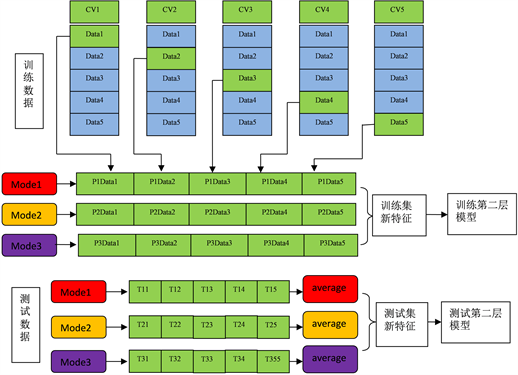Figure 3. The schematic diagram of stacking method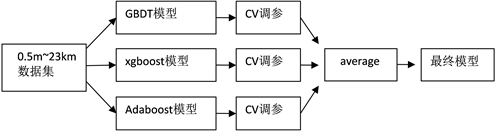Figure 4. The flow chart of average model

Average融合的算法相对于stacking较为简单，它只是将所有基模型的输出结果取平均后作为最终结果。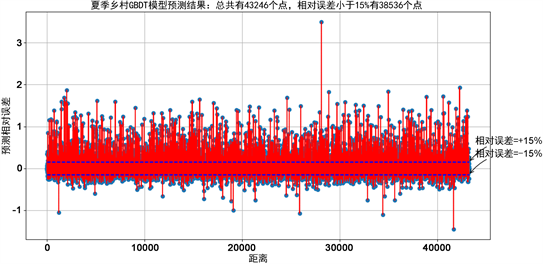Figure 5. The error of GBDT model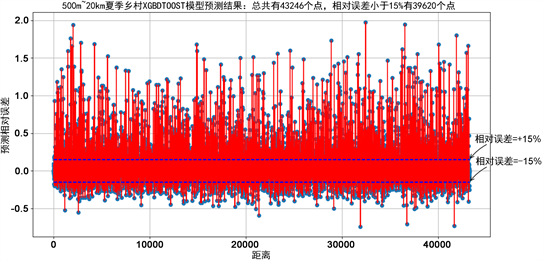Figure 6. The error of xgboost model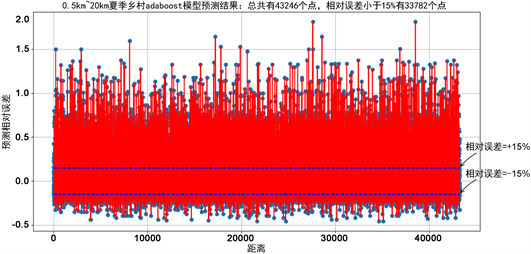Figure 7. The error of adaboost model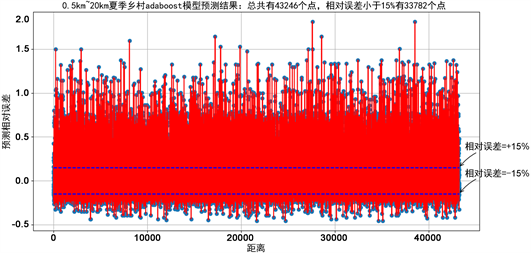Figure 8. The error of stacking model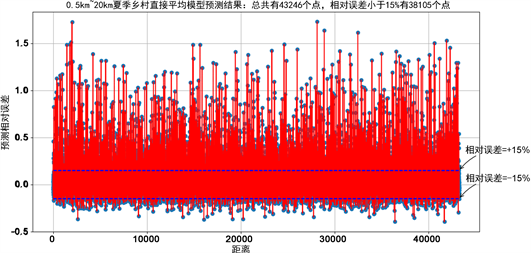Figure 9. The error of average modelTable 2. The statistical characteristics of the five models’ predicting error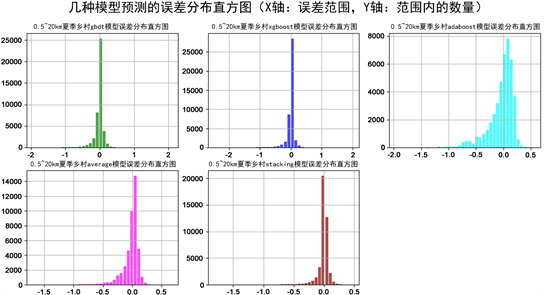231：单gbdt模型预测误差分布；232：单xgboost模型预测误差分布；233：单adaboost预测误差分布；233：average融合的误差分布；234：stacking融合的误差分布。

Figure 10. The histogram of the five models’ predicting error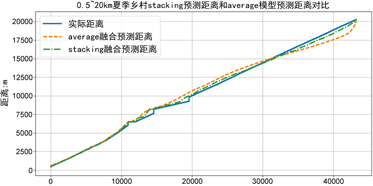Figure 11. The comparison of stacking and average model

6. 结论

Passive IR Ranging Based on Ensemble Learning[J]. 计算机科学与应用, 2019, 09(03): 604-612. https://doi.org/10.12677/CSA.2019.93068

1. 1. 赵洪宇, 何建伟, 王卫红. 针对海面目标的机载红外单站几何定位技术[J]. 中国电子科学研究院学报, 2017, 12(4): 410-413.

2. 2. 李盈, 马宁, 贾鹏, 等. 机载远程红外双站测距研究[J]. 激光与红外, 2017, 47(8): 982-986.

3. 3. 杨德贵, 黎湘, 肖顺平. 基于单站双波段红外图像的面目标被动测距研究[J]. 信号处理, 2010, 26(5): 714-718.

4. 4. 安永泉, 王志斌, 李晋华, 等. 基于O2吸收特性单站被动测距机理研究[J]. 红外与激光工程, 2015, 44(1): 310-316.

5. 5. 飞行器尾焰红外辐射及其被动测距[J]. 红外与激光工程, 2013, 42(7): 1660-1664.

6. 6. 冯国强, 邹强, 李伟仁. 单站双波段红外被动测距算法研究[J]. 红外技术, 2005, 27(4): 295-298.

7. 7. Drucker, H. (1997) Improving Regressors Using Boosting Techniques. In: 14th International Con-ference on Machine Learning, Morgan Kaufmann Publishers Inc., 107-115.

8. 8. Friedman, J., Hastie, T. and Tibshirani, R. (2000) Additive Logistic Regression: A Statistical View of Boosting (With Discussion and a Rejoinder by the Authors). Annals of Statistics, 28, 337-374. https://doi.org/10.1214/aos/1016218223

9. 9. Friedman, J.H. (2001) Greedy Function Approximation: A Gradient Boosting Machine. Annals of Statistics, 29, 1189-1232. https://doi.org/10.1214/aos/1013203451

10. 10. Freund, Y. and Schapire, R.E. (1995) A Decision-Theoretic Generalization of On-Line Learning and an Application to Boosting.

11. 11. Chen, T. and Guestrin, C. (2016) XGBoost: A Scalable Tree Boosting System. In: ACM SIGKDD International Conference on Knowledge Discovery and Data Mining, ACM, New York, 785-794.

12. 12. 马鹤. 基于MODTRAN的复杂大气条件下红外系统作用距离计算[D]: [硕士学位论文]. 南京: 南京航空航天大学, 2013.

13. 13. Wang, S.Q., Yang, J. and Chou, K.C. (2006) Using Stacked Generalization to Predict Membrane Protein Types Based on Pseudo-Amino Acid Composition. Journal of Theoretical Biology, 242, 941. https://doi.org/10.1016/j.jtbi.2006.05.006

14. 14. Wolpert, D.H. (1992) Stacked Generalization. Neural Networks, 5, 241-259. https://doi.org/10.1016/S0893-6080(05)80023-1

15. 15. Freund, Y. (1995) Boosting a Weak Learning Algorithm by Majority. In-formation & Computation, 121, 256-285. https://doi.org/10.1006/inco.1995.1136

16. NOTES

*通讯作者。